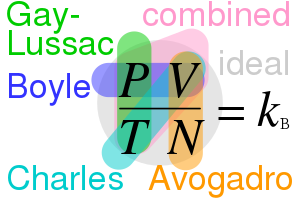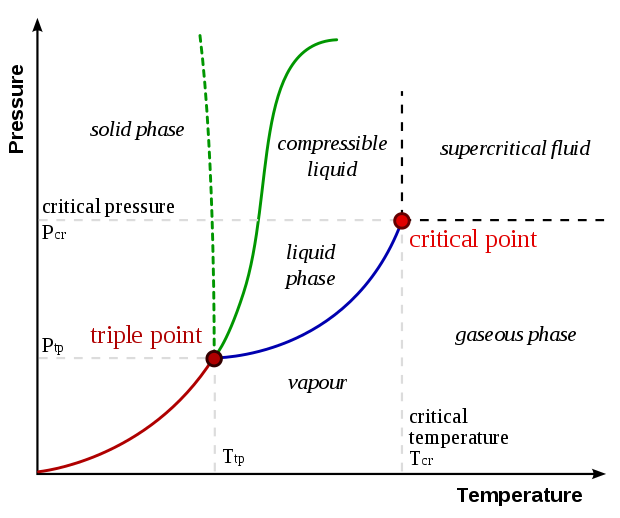17: Temperature, Thermal Expansion, & Ideal Gas Law

# 17.1: Atomic Theory #

• Atoms are the smallest unit of matter
• Atomic unit: .$\text{u} = 1.66\cdot 10^{-27}$ kg
• E.x. Hydrogen weighs .$1.0078 \text{u}$
• Molecular mass of a compound is the sum of the particles (atoms) in the compound

## Terms #

• Element: Substance that cannot be broken down into smaller substances (gold)
• Molecule: Group of atoms held together by covalent bonds
• Compound: Substance made from atoms combined in specific ratios

## Brownian Motion #

• Random movement seen in pollen/dust, as well as atoms
• Using Brownian motion, Einstein found the size of an atom to be .$10^{-10}$ meters

## Forces #

• Atoms and molecules exert an (electric) attractive force on one another by default
• If an atom/molecule gets too close to another, they exert a repelling force on one another
• Matter states:
• Solid:
• Atoms held in matrix formation by strong attractive forces.
• Atoms vibrate around their mean position
• Liquid:
• Force between atoms is weaker so atoms move more rapidly within
• Gas:
• Atom attractive forces are so weak compared to their kinetic energy that they move randomly
• If two atoms collide, the attractive force is so weak that they may just bounce off one another

# 17.2: Temperature and Thermometers #

• Matter property changes under different temperatures
• Sidewalks expand under the sun
• Electric resistance increases with heat
• Lightbulb filament glows

## Thermometers Types #

• Originally used alcohol which expands linearly with heat (water doesn’t)
• Bimetalic strips bend at slightly different rates under heat
• Electronic thermometers measure resistance change and often have digital screens

## Scales #

• Fahrenheit: Water freezes at 32 and boils at 212 deg
• Celsius: Water freezes at 0 and boils at 100 deg
• Kelvin: Celsius + 273.15K. Written without degree sign. Absolute = 0K
• Conversions: $$T(^\circ C) = \frac{5}{9}(T(^\circ F)-32)$$ $$T(^\circ F) = \frac{9}{5}(T(^\circ C)) + 32$$
• Different materials expand at different rates ro we use constant-volume thermos because it’s pressure linearly relates to the temperature

# 17.3 0th Law of Thermodynamics #

• If objects .$A$ and .$B$ are at equilibrium with object .$C$ , then .$A$ and .$B$ are also at equilibrium with one another
• Systems naturally reach equilibrium over time

## Thermal Expansion #

• Most materials expand when heated
• Expansion amount depends on the material
• Equations (assuming a constant volume .$V$ )
• Linear Expansion:
• .$\alpha$ is the coefficient of linear expansion and depends on the material with units .$(^\circ C)^{-1}$ $$\Delta l \approxeq \alpha l_0 \cdot \Delta T$$ $$l_i + \Delta l = l_f = l_i ( 1 + \alpha\Delta T)$$ $$\frac{dl}{dT} = \alpha(T)\cdot l$$
• If .$\Delta T$ is too large such that the temperature dependence of .$\alpha$ is too large, we can do the following: $$\int_{l_i}^{l_f} \frac{1}{l}dl = \int_{T_i}^{T_f} \alpha(T) dT$$
• Volume Expansion: $$\beta = \frac{1}{V} \frac{dV}{dT}$$ $$V_f \approxeq V_0 ( 1 + \beta\Delta T)$$
• .$\beta \approx 3\cdot\alpha$ = coefficient of volume expansion.
• Coefficient of expansion varies at extremely high heats so it only works with small .$\Delta T$ ’s
• Materials must be isotropic (have same expansion properties in all directions) for us to say .$\alpha \approx 3\cdot\beta$
• (Linear) expansion doesn’t exist for gas or liquids because they have no fixed space like solids.
• Weird water property
• .$0 - 4 ^\circ C$ : Water increases in density .$\rho^+\Longrightarrow$ decreases in volume .$V^-$
• .$4^\circ C +$ : Water acts “normally”: increase in volume .$V$ proportional to temperature .$T$
• This explains why pipes burst when frozen and why ice cubes float

# 17.5 Thermal Stresses #

• When the ends a solid (rod) are fixed (such as in beams), temperature changes induce thermal stress due to the clamp limiting expansion/contraction
• Process Steps:
1. Beam tries to expand/contract by .$\Delta l$
2. Mount reacts with an opposite reactive force, keeping it at it’s original length: $$\Delta l = \frac{1}{E} \cdot \frac{F}{A} \cdot l_0$$ where .$E$ is Young’s modulus for the material. We can also re-write for stress: $$\frac{F}{A} = \Delta l \cdot E \cdot \frac{1}{l_0} = (\alpha l_0 \Delta T) E \cdot \frac{1}{l_0} = \alpha E \Delta T$$

# 17.6 Gas Laws and Absolute Temperature #

• Equation at State describes how pressure varies with Temperature, Number of Particles (Molecules), and Volume
• State is the physical condition of a system
• Equilibrium State: .$T, N, \&\ V = \text{Constant}$

## Laws #

• Assume that gasses aren’t too dense (so .$P \sim$ atmospheric pressure) and that they aren’t close to liquefaction (boiling) point either (for oxygen, this is .$~183^\circ \text{C}$.)• Boyle’s Law
• .$V \propto P^{-1}$ [Constant Temperature]
• .$P$ is absolute, not gauge, pressure
• Alternatively, .$PV =$ const or .$P_1V_1 = P_2V_2$
• Charles’s Law
• .$V \propto T$ [Constant Pressure]
• Alternatively, .$\frac{V_1}{T_1} = \frac{V_2}{T_2}$
• Gay Lussac’s Law
• .$P \propto T$ [Constant Volume]
• Alternatively, .$\frac{P_1}{T_1} = \frac{P_2}{T_2}$

# 17.7 Ideal Gas Law #

$$PV = nRT = n k_B N_a T = N k_B T$$

• .$P$ is the pressure of the gas [Pascals]
• .$V$ is the volume of the gas [Cubic Meters]
• .$T$ is the absolute temperature of the gas [Kelvins]
• .$N$ is the number of molecules of gas
• .$n$ is the amount of substance of gas (number of moles) [Moles]
• .$R$ is the ideal, or universal, gas constant, equal to .$k_B \cdot N_a = 8.314 \frac{J}{K\cdot \text{mol}}$
• Using mass of a gas, different gasses have different proportionality constants
• So we used number of moles, in which case .$R$ becomes the constant for all gasses
• .$k_B$ is the Boltzmann constant
• Relates the average relative kinetic energy of particles in a gas with the thermodynamic temperature of the gas
• .$N_a$ is the Avogadro constant
• The number of particles that are contained in one mole of gas
• .$n = N/N_A$
• This equation is Ideal in that the equation only works for gasses around atmospheric pressure and not excessive temperatures

## Moles #

• Mole is the SI unit for amount of substance
• 1 mole = Number of particles in .$\text{12g}$ of Carbon
• 1 mole = Number of grams of a substance numerically equal to the molar mass $$n \text{(moles)} = \frac{\text{mass (grams)}}{\text{molecular mass (g/mol)}}$$

# 17.8 Problem Solving with .$PV = nRT$ #

## STP: Standard Temperature and Pressure #

• .$T = 273 \text{K}$
• .$P = 1.00 \text{atm} = 1.013\cdot10^5 \text{N/m}^2 = 101.3 \text{kPA}$
• .$1 \text{mol of ideal gas} = 22.4\text{L}$ in volume
• If P is in liters and V is in atm, then we can use .$R = 0.0821 \frac{\text{L} \cdot \text{atm}}{\text{mol} \cdot \text{K}}$
• Since .$n$ and .$R$ are constants, we can say: $$\frac{P_1 V_1}{T_1} = \frac{P_2 V_2}{T_2}$$

# 17.9 Ideal Gas with Avogadro’s Number #

• Equal volume of gas with the same .$P$ and .$T$ have an equal .$n$ umber of particles (molecules)
• .$N_a$ is avogadro’s number: the number of particles that are contained in one mole of gas (or one gram of hydrogen).
• .$N_a = 6.022 \cdot 10^{23}\ \text{particles/mole}$
• Therefore, if .$N$ is the number of molecules of a gas sample and .$n$ is the number of moles, then $$N = n\cdot N_A \Longrightarrow n = \frac{N}{N_A} \Longrightarrow PV = \frac{N}{N_A}RT = Nk_B T$$ where .$k_B$ is Boltzmann’s constant .$\frac{R}{N_A} = 1.38 \cdot 10^{-23} \frac{\text{J}}{\text{K}}$
• .$P_3 = 4.88\ \text{torr};\ T_3 = 0.01^\circ C$ for water
• Ideal Gas, constant volume: $$T = (273.16 K)\bigg(\frac{P}{P_3}\bigg)$$
• Constant volume: $$T = (273.16 K)\lim_{P_3 \to 0}\bigg(\frac{P}{P_3}\bigg)$$A typical phase diagram. The solid green line applies to most substances; the dashed green line gives the anomalous behavior of water. For more see 18.4